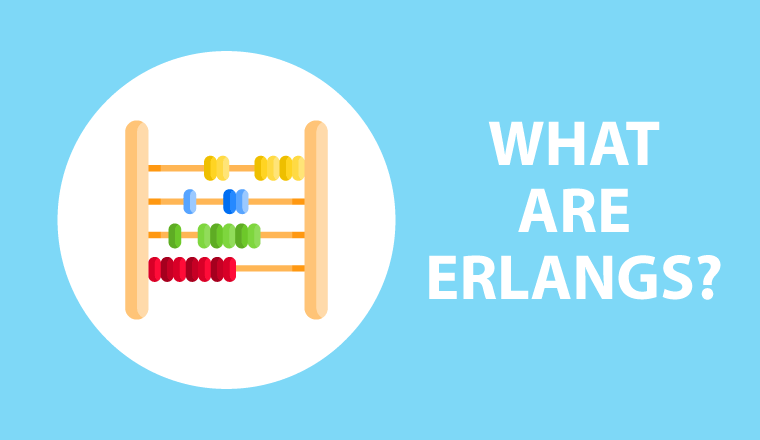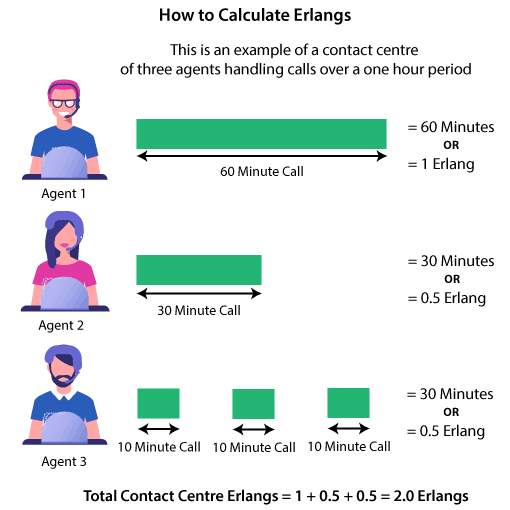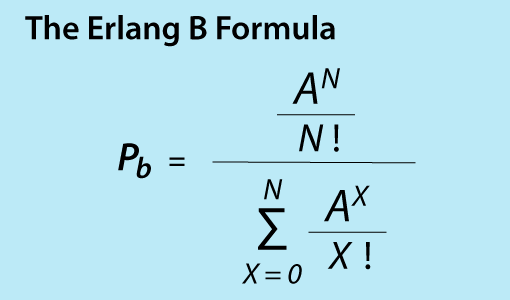# What are Erlangs?﻿
10,703

We define what an Erlang is before sharing why and how they are used in the contact centre.

## Erlang Definition

The simplest definition of an Erlang is that it is a measure of telecommunications usage.

While Erlang is also a computer programming language, the term more commonly refers to the telecommunications measure which plays a big role in many contact centre calculations.

One Erlang would equate to one hour’s worth of phone calls…

One Erlang would equate to one hour’s worth of phone calls, as standardized by the International Telecommunication Union (ITU).

So, if you have a phone contract of 60 minutes, you have effectively purchased one Erlang’s worth of telephone calls.

Another example would be if your contract is 240 minutes long, that means that you have four Erlangs of telephone calls available to use (240 minutes of calls = 4 Erlangs).

If you are, however, looking for a more technical definition, this is ITU’s definition:

“A unit of traffic intensity. It is a dimensionless quantity expressing voice activity in units of time that would be seen during some time intervals, typically an hour. It is used to determine the number of circuits need to satisfy circuit voice demand.”

## Applying This to the Contact Centre

One Erlang in the contact centre would equate to 60 minutes of calls taken by the contact centre team.

The following graphic gives a good representation of this principle:So, if you were to collate the number of minutes that your team handled within a certain time period, and divide that number by 60, you would have the number of Erlangs for that time interval.

While this may at first seem like a frivolous task to undertake, it does have a few great uses in the contact centre.

## Why Do Erlangs Matter?

In the past, Erlangs were most commonly used as part of the Erlang B equation.The Erlang B equation enables you to work out how many telephone lines you need coming into a building to support a given contact volume of calls.

More to the point, the Erlang B equation would enable you to calculate how many lines you would need to allow for queuing.

By using the Erlang B equation alongside forecast call volumes and Average Handle Time (AHT), we can also predict how quickly on average the queue would move.

This final Erlang B calculation still matters for contact centres, while calculating the number of telephone lines is becoming less important in today’s digital world.

However, as time has passed, the great potential of Erlangs is being recognized.

Now, most contact centres are using Erlangs for staffing calculations and predicting their abandon rate, making it a very valuable contact centre measure, as explained in more detail below.

## How Are Contact Centres Now Using Erlangs?

While we have earlier focused on the Erlang B formula, the modern contact centre will likely concentrate much more on the Erlang C and Erlang A formulas.

The Erlang C formula is the equation that sits behind an Erlang Calculator. An Erlang Calculator enables you to determine how many contact centre agents you need to answer an estimated call volume – while also meeting service level and occupancy targets.

It also enables you to calculate the probability that a call will have to be queued, based on the expected call volumes and number of agents available.

The Erlang A formula is an extension of the Erlang C formula and uses Erlangs to estimate abandoned calls, alongside a metric called average patience.

To find out how to use the Erlang A formula to estimate caller abandons and why this is important, read our article: A Beginner’s Guide to the Erlang A Formula

## So, Where Did Erlangs Come From?

While Erlangs have all of these great uses in the modern contact centre, it’s a surprisingly old measure.

In fact, it was over 100 years ago when Danish mathematician A.K. Erlang first developed the Erlang C formula in 1917, alongside his new measure of telecommunications usage: the Erlang.

Danish mathematician A.K. Erlang first developed the Erlang C formula in 1917, alongside his new measure of telecommunications usage: the Erlang.

Of course, contact centres weren’t around at this time – with the first British contact centre not coming into existence until 1965, according to our article: The History of the Call Centre – Updated

So, A.K. Erlang would have likely been shocked to see how his formula, first put forward in his paper “Solution of some problems in the theory of probabilities of significance in automatic telephone exchanges”, is still being used to such a great effect so many years later.

However, Erlang can’t take all the credit for all of the formulas, as it was Swedish mathematician Conny Palm who created the Erlang A formula in 1946. However, Erlangs were of course a key input into this equation too!

## In Summary

Erlangs are a simple measure of telecommunications usage, with every 60 minutes of phone calls equating to one Erlang.

Erlangs are used as inputs into three key equations, which are known as the Erlang A, Erlang B and Erlang C formulas.

These equations can all be utilized by contact centres to great effect, the most useful being the Erlang C in enabling you to calculate how many staff  you need in a contact centre.

For more on these three key Erlang formulas, read our articles:

Author: Robyn Coppell